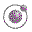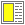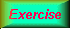Expressions, Types, and Functions - slide 20 : 46

Practical list construction
cons is the basic list constructor function - but it can be applied through a number of other means as well
• List and S-expression construction:

• Deep cons expressions

• Using the list function

• Using quote or quasiquote also known as backquote

 Expression Value `(cons 1 (cons 2 (cons (+ 1 2) '())))` `(1 2 3)` `(list 1 2 (+ 1 2))` `(1 2 3)` `(quote (1 2 (+ 1 2)))` `(1 2 (+ 1 2))` `'(1 2 (+ 1 2))` `(1 2 (+ 1 2))` `(quasiquote (1 2 (unquote (+ 1 2))))` `(1 2 3)` ``(1 2 ,(+ 1 2))` `(1 2 3)`Every second element of a list